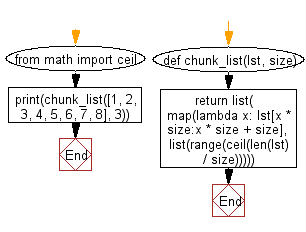﻿ Python: Chunk a given list into smaller lists of a specified size - w3resource

# Python: Chunk a given list into smaller lists of a specified size

## Python List: Exercise - 232 with Solution

Write a Python program to chunk a given list into smaller lists of a specified size.

• Use list() and range() to create a list of the desired size.
• Use map() on the list and fill it with splices of the given list.
• Finally, return the created list.

Sample Solution:

Python Code:

``````from math import ceil
def chunk_list(lst, size):
return list(
map(lambda x: lst[x * size:x * size + size],
list(range(ceil(len(lst) / size)))))
print(chunk_list([1, 2, 3, 4, 5, 6, 7, 8], 3))
```
```

Sample Output:

```[[1, 2, 3], [4, 5, 6], [7, 8]]
```

Flowchart:## Visualize Python code execution:

The following tool visualize what the computer is doing step-by-step as it executes the said program:

Python Code Editor:

Have another way to solve this solution? Contribute your code (and comments) through Disqus.

What is the difficulty level of this exercise?

Test your Programming skills with w3resource's quiz.

﻿

## Python: Tips of the Day

Returns True if the provided function returns True for every element in the list, False otherwise:

Example:

```def tips_every(lst, fn=lambda x: x):
return all(map(fn, lst))

print(tips_every([2, 4, 3], lambda x: x > 1))
print(tips_every([1, 2, 3]))
```

Output:

```True
True
```